# matlab拟合工具箱

x=1:10;
y=randn(size(x));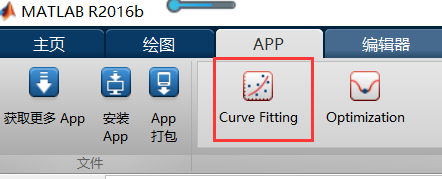>> cftool


• SSE，误差平方和，The sum of squares due to error，即最小二乘
S S E = ∑ i = 1 n ( y ^ i − y i ) 2 SSE=\sum_{i=1}^n(\hat y_i- y_i)^2

• MSE， mean squared error， 均方误差，即误差的平方和的均值，就等于SSE除以n，n为数据点个数

• RMSE，Root mean squared error，均方根误差，也叫回归系统的拟合标准差，等于MSE开方

• R-square，Coefficient of determination，确定系数

SSR: Sum of squares of the regression，即预测数据与原始数据均值之差的平方和
S S R = ∑ i = 1 n ( y ^ i − y ˉ ) 2 SSR=\sum_{i=1}^n(\hat y_i-\bar y)^2

SST: Total sum of squares，即原始数据均值之差的平方和
S S T = ∑ i = 1 n ( y i − y ˉ ) 2 SST=\sum_{i=1}^n( y_i-\bar y)^2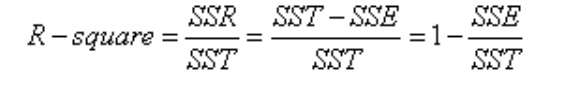S S R + S S E = S S T SSR+SSE=SST
“确定系数”是通过数据的变化来表征一个拟合的好坏。

“确定系数”的正常取值范围为[0 1]，越接近1，表明方程的变量对y的解释能力越强，这个模型对数据拟合的也较好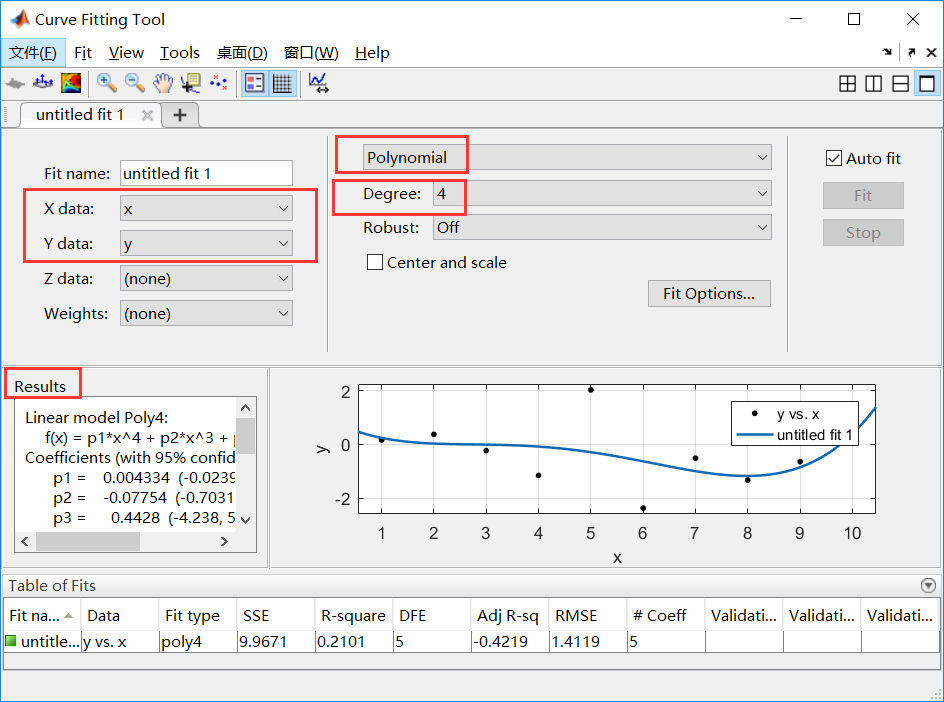可以选择各种拟合方式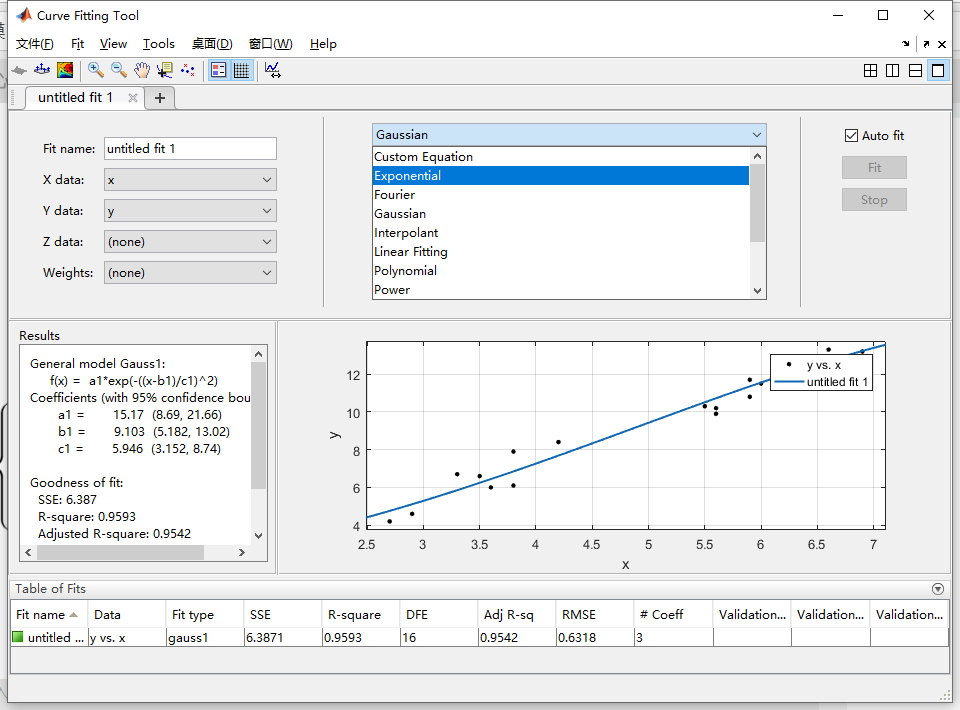# 最小二乘拟合

## 理论推导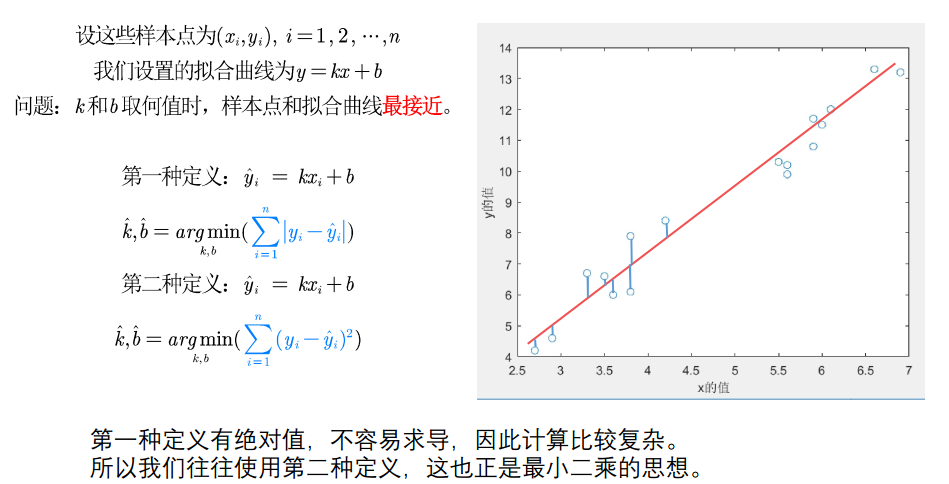## 用最小二乘法求解线性回归的k,b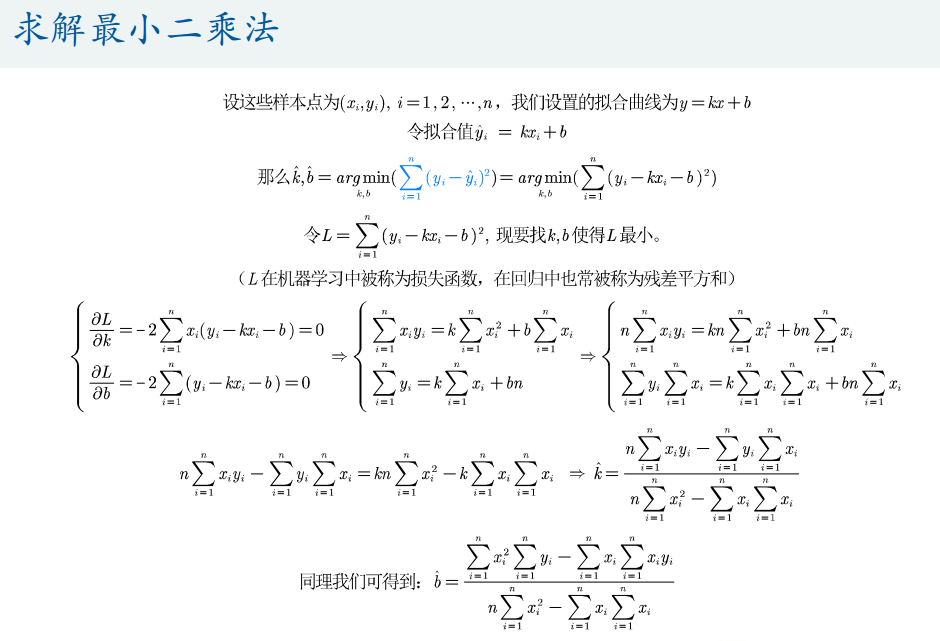## 怎么评价拟合的精度

SSE是我们最容易想到的，每个拟合值和准确值的差值平方和，但是对于线性拟合，还可以引入SSR和SST

SSE当然是越小越好

SST是原值和均值的距离平方和，正比于数据方差，衡量的是原始数据的偏离程度

SSR是拟合值和原始数据均值的距离平方和，衡量拟合数据的偏离程度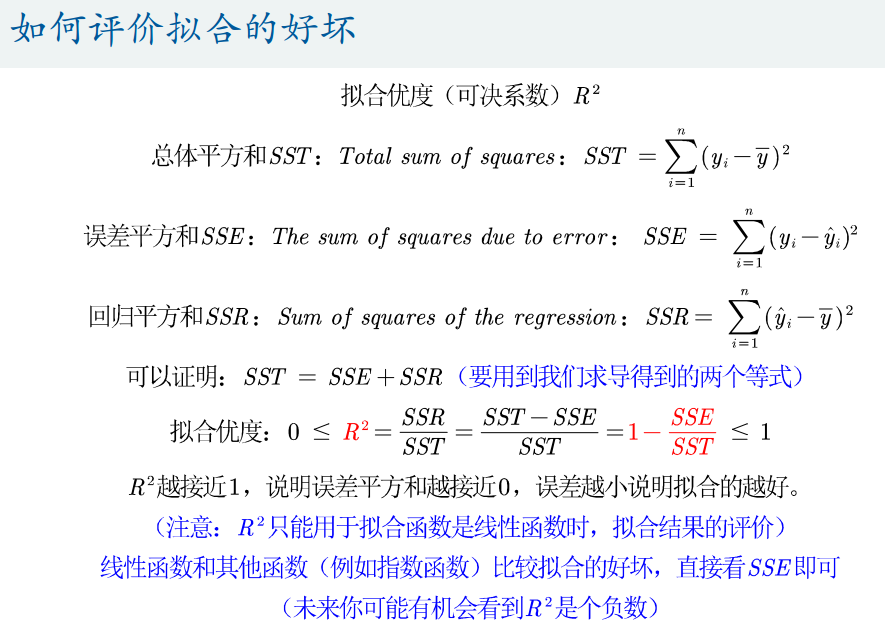R 2 R^2 是负数的例子来了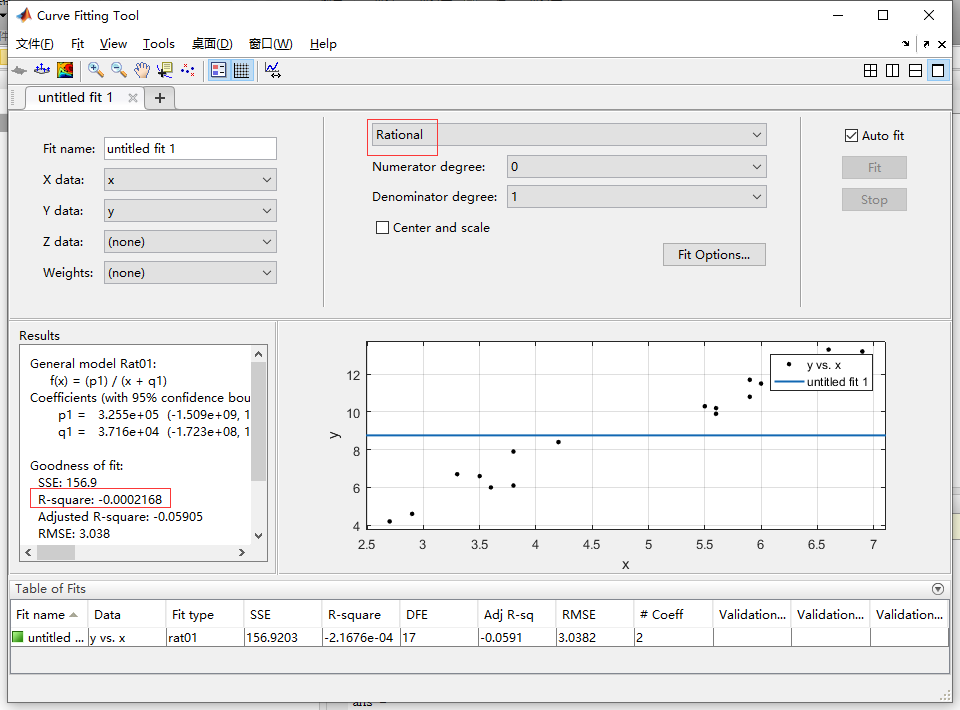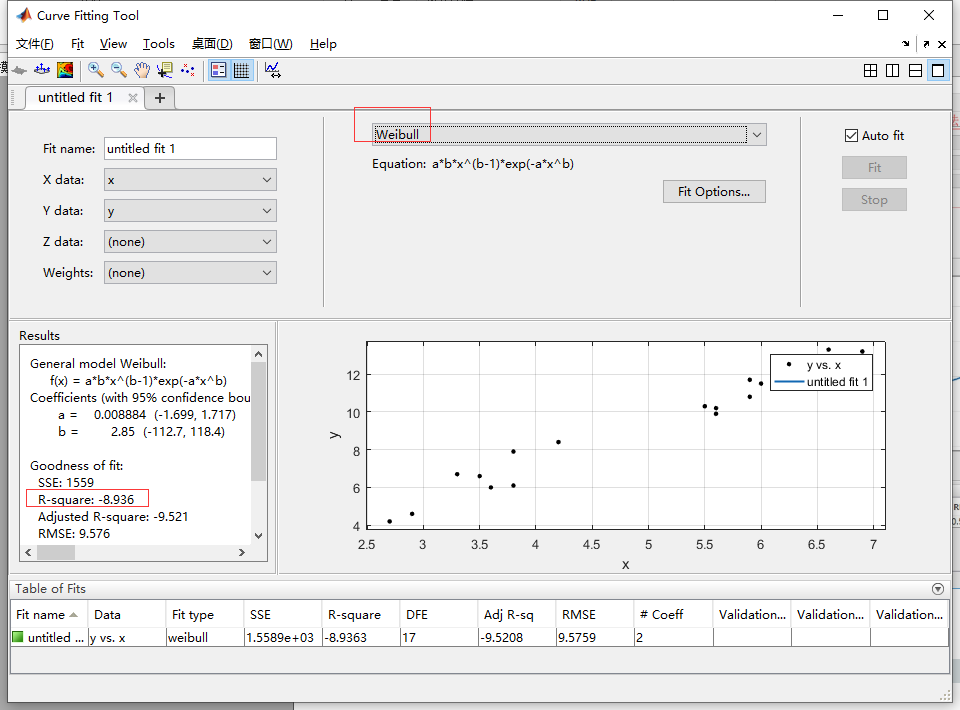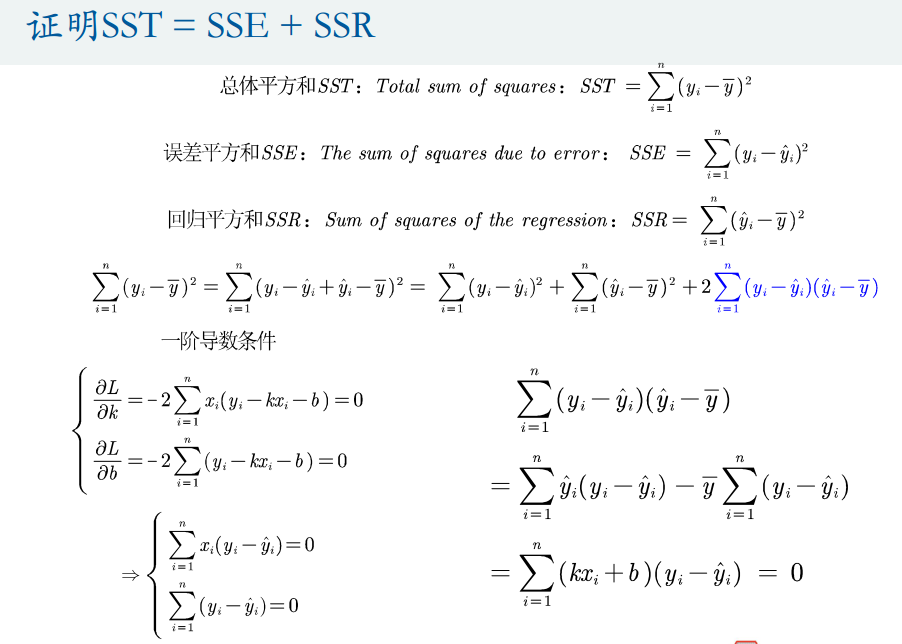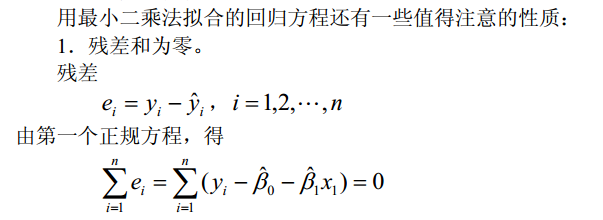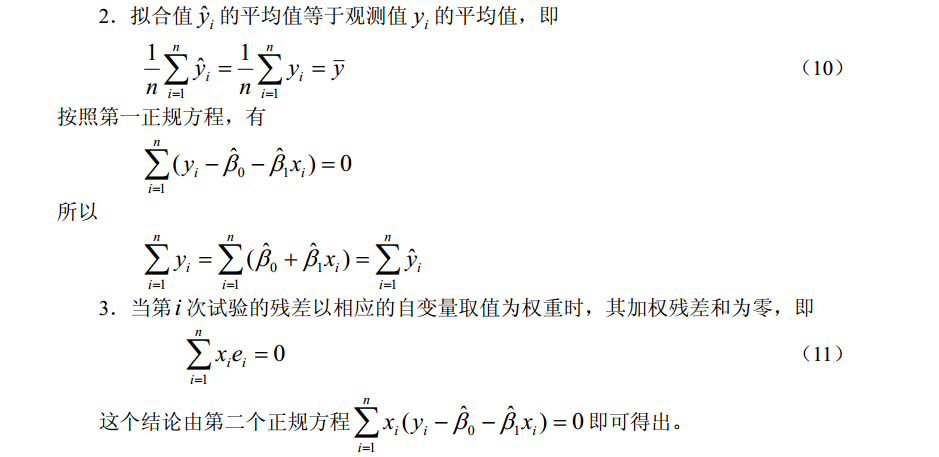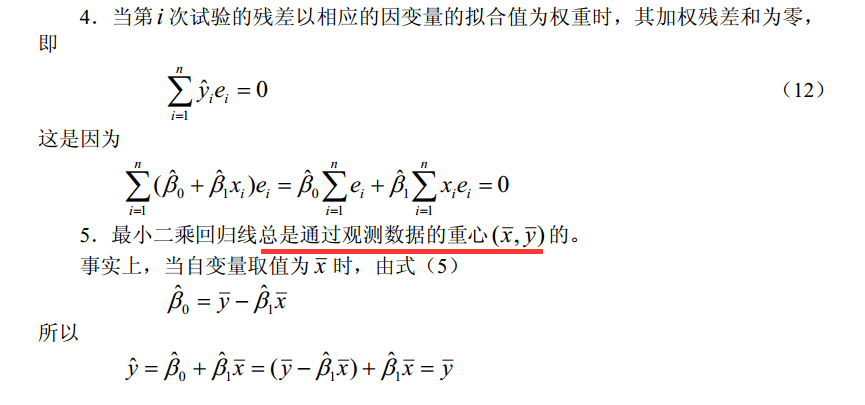## 一个例子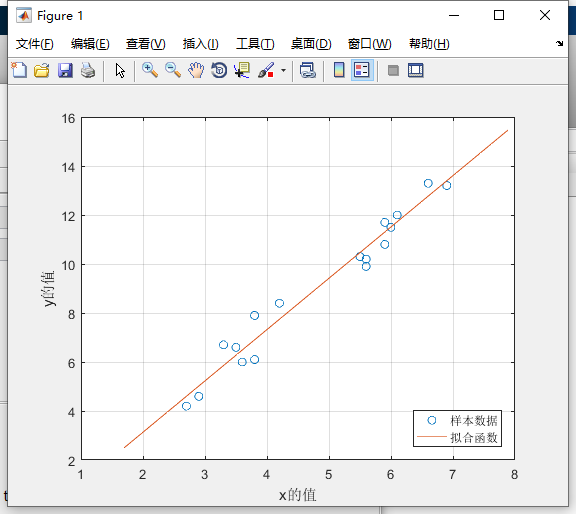clear;clc
plot(x,y,'o')
% 给x和y轴加上标签
xlabel('x的值')
ylabel('y的值')
n = size(x,1);
k = (n*sum(x.*y)-sum(x)*sum(y))/(n*sum(x.*x)-sum(x)*sum(x))
b = (sum(x.*x)*sum(y)-sum(x)*sum(x.*y))/(n*sum(x.*x)-sum(x)*sum(x))
hold on % 继续在之前的图形上来画图形
grid on % 显示网格线

% % 画出y=kx+b的函数图像 plot(x,y)
% % 传统的画法：模拟生成x和y的序列，比如要画出[0,5]上的图形
% x = 0: 0.1 :5  % 间隔设置的越小画出来的图形越准确
% y = k * x + b  % k和b都是已知值
% plot(x,y,'-')

f=@(x) k*x+b;
fplot(f,[min(x)-1,max(x)+1]);
legend('样本数据','拟合函数','location','SouthEast')

% 匿名函数的基本用法。
% handle = @(arglist) anonymous_function
% 其中handle为调用匿名函数时使用的名字。
% arglist为匿名函数的输入参数，可以是一个，也可以是多个，用逗号分隔。
% anonymous_function为匿名函数的表达式。
% 举个小例子
% % >> z=@(x,y) x^2+y^2;
% % >> z(1,2)
% % ans =  5
% fplot函数可用于画出匿名函数的图形。
% fplot(f,xinterval) 将在指定区间绘图。将区间指定为 [xmin xmax] 形式的二元素向量。

y_hat = k*x+b; % y的拟合值
SSR = sum((y_hat-mean(y)).^2)  % 回归平方和
SSE = sum((y_hat-y).^2) % 误差平方和
SST = sum((y-mean(y)).^2) % 总体平方和
SST-SSE-SSR  % 非常小的数，接近0
R_2 = SSR / SST % 拟合优度


## 另一个例子，薄膜渗透率题目，最小二乘拟合溶液浓度变化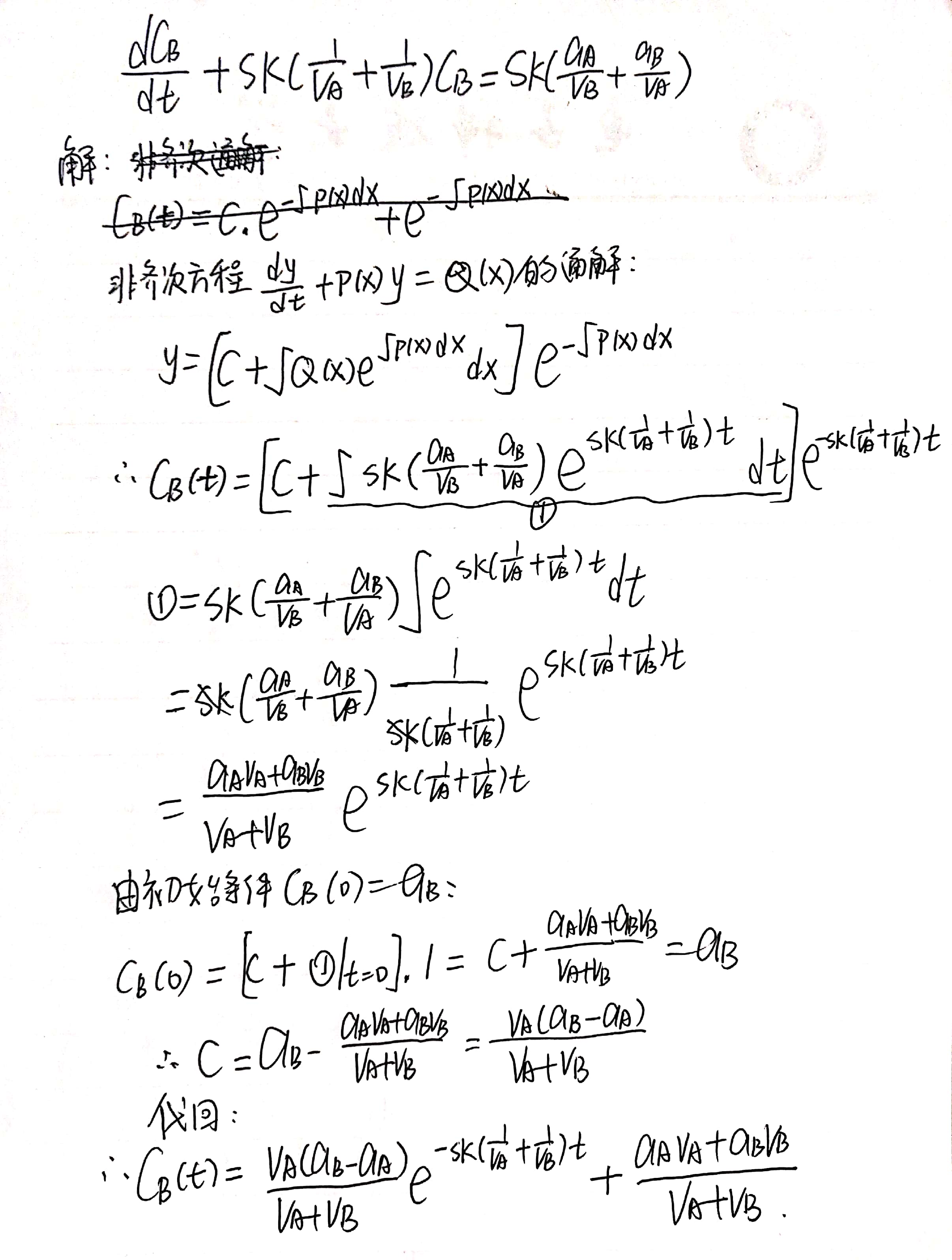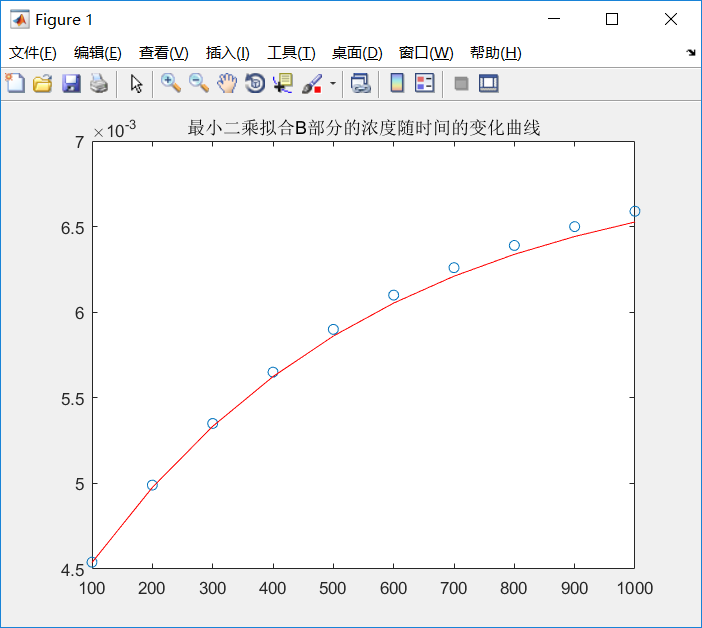clear all
clc
tdata=linspace(100,1000,10); % 时间，秒
cdata=1e-05.*[454 499 535 565 590 610 626 639 650 659];% 浓度测量值，mg/cm3转化为g/cm3，再去掉小数点
x0=[0.2 0.05 0.08]; % 随便设置最小化问题的三个参数的初始值
x=lsqcurvefit('curvefun',x0,tdata,cdata) % least square ，最小二乘曲线拟合
% 第一个参数是被优化的函数，x0是初始参数，后面是优化依靠的实际数据，返回一组参数使得数据点和函数计算值的误差平方和最小
f=curvefun(x,tdata);
plot(tdata, cdata,'o',tdata,f,'r-')
title('最小二乘拟合B部分的浓度随时间的变化曲线')

% curvefun.m
function f=curvefun(x,t);
f=x(1)+x(2)*exp(-0.02*x(3)*t); % x(1),x(2),x(3)分别是a,b,k


# 多项式拟合

x=1:10;
y=randn(size(x));
p=polyfit(x,y,7); % 7阶多项式，返回拟合多项式的降幂系数
x1=0:.1:10;
y_hat=polyval(p,x1);
plot(x1,y_hat,x,y,'r*')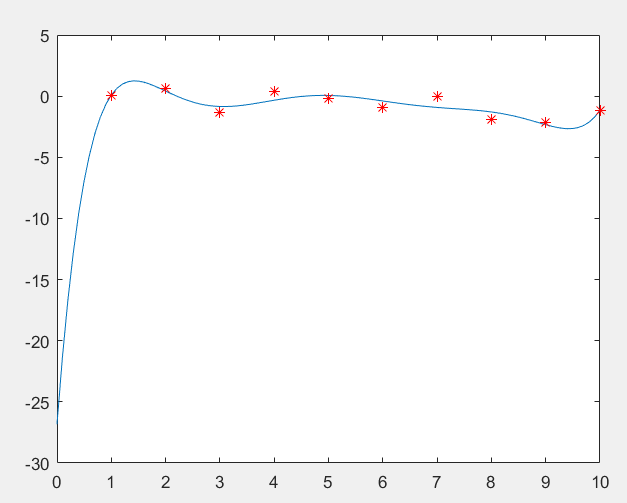# 自定义函数拟合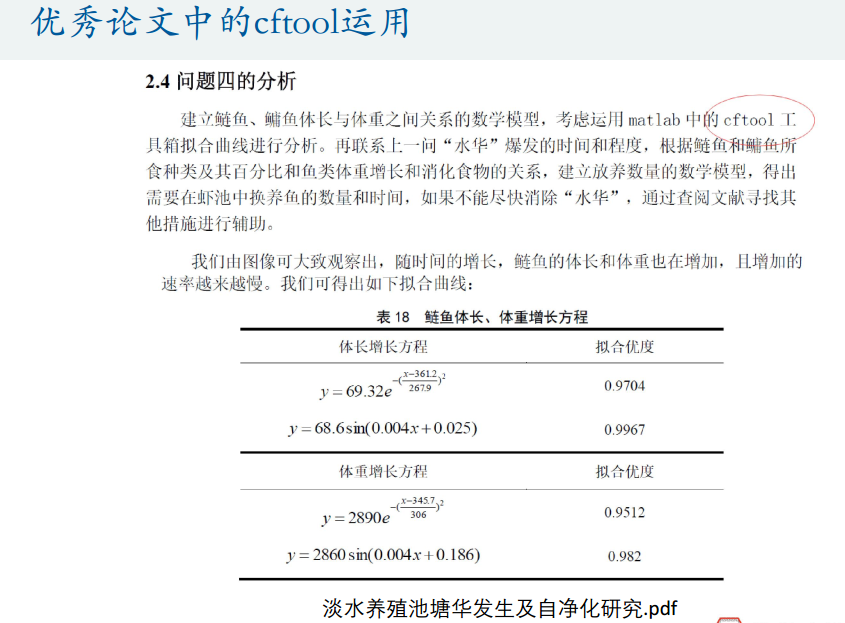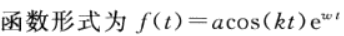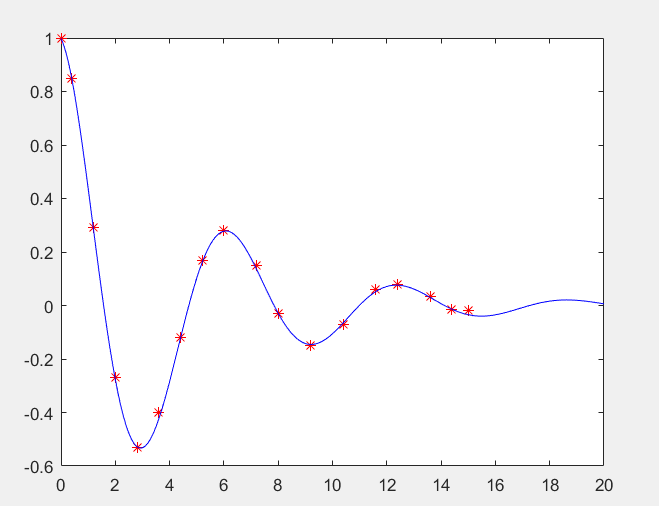syms t
x=[0 0.4 1.2 2 2.8 3.6 4.4 5.2 6 7.2 8 9.2 10.4 11.6 12.4 13.6 14.4 15];
y=[1 0.85 0.29 -0.27 -0.53 -0.4 -.12 .17 .28 .15 -.03 -.15 -.071 .059 .08 .032 -.015 -.02];
x=x';y=y';
% 自定义拟合函数
f=fittype('a * cos(k * t) * exp(w * t)','independent','t','coefficients',{'a','k','w'});
cfun=fit(x,y,f); % 数据必须以列向量形式给出
x1=0:.1:20;
y1=cfun(x1);
plot(x,y,'r*',x1,y1,'b-')


cfun函数还给了每个参数的置信区间！！简直完美

警告: Start point not provided, choosing random start point.
> In curvefit.attention.Warning/throw (line 30)
In fit>iFit (line 299)
In fit (line 108)
In me_test (line 8)
>> cfun
cfun =
General model:
cfun(t) = a * cos(k * t) * exp(w * t)
Coefficients (with 95% confidence bounds):
a =      0.9987  (0.9836, 1.014)
k =       1.001  (0.9958, 1.006)
w =     -0.2066  (-0.2131, -0.2002)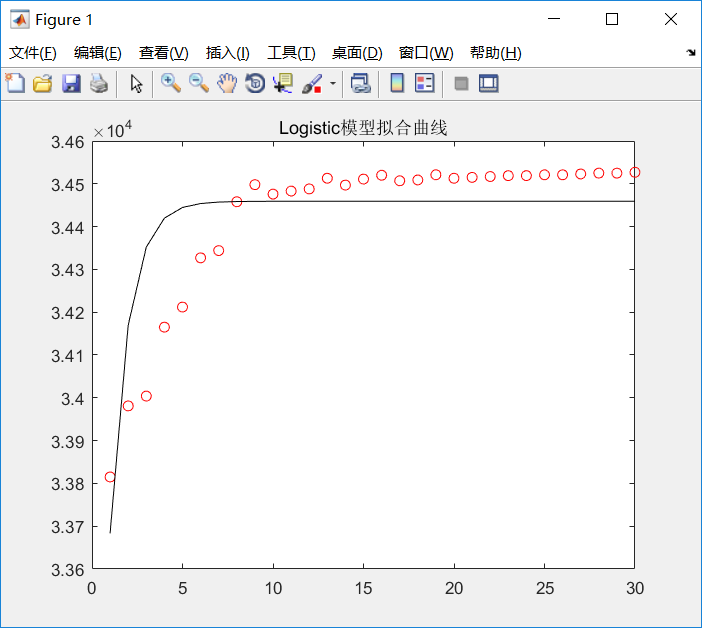clear all
clc
% 人口数据
T=1:30;  % +1970=年份
P=[33815 33981 34004 34165 34212 34327 34344 34458 34498 34476 34483 34488 34513 34497 34511 34520 34507 34509 34521 34513 34515 34517 34519 34519 34521 34521 34523 34525 34525 34527];%人口
%plot(T,P)% 折线
plot(T,P,'r o')% 只画数据点
hold on
% 线性化处理
for t=1:30
x(t)=exp(-t);
y(t)=1/P(t);
end

c=zeros(30,1)+1;
X=[c,x'];
B=inv(X' * X) * X' * y' % 线性回归的回归系数，2行1列，B（1,1）是0次幂系数，B（2,1）是1次幂系数
for i=1:30
z(i)=B(1,1)+B(2,1)*x(i) ; % 回归拟合值
s(i)=y(i)-sum(y)/30 ;  % 离差，每个数据和平均值的距离
e(i)=z(i)-y(i);  % 拟合误差
end
SST=s*s' ; % 离差平方和
SSE=e*e' ; % 误差平方和
SSR=SST-SSE;  % 预测数据和原始数据均值的平方和
F=28*SSR/SSE  % F检验值

% 计算非线性回归模型的拟合值
for j=1:30
Y(j)=1/(B(1,1)+B(2,1)*exp(-j));
end
% 画出逻辑函数拟合曲线
plot(T,Y,'k ')
title('Logistic模型拟合曲线')


F检验值？？？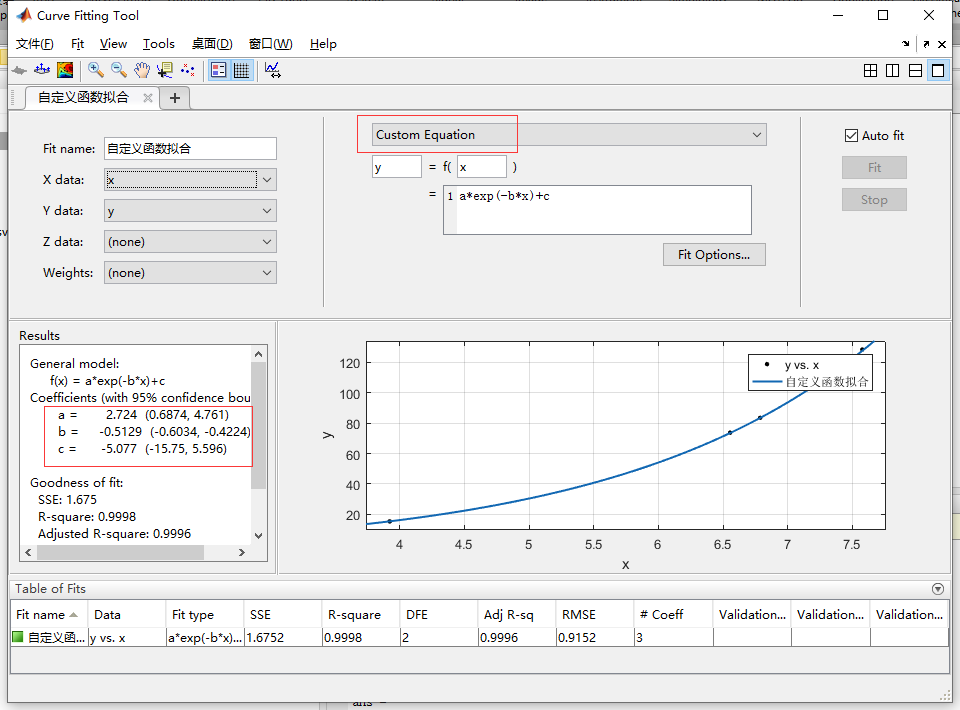% a + (b-a) * rand(1,5); 如：
a= 0;
b=10;
x= a + (b-a) * rand(1,5); % 1*5的随机矩阵
e=normrnd(0,1,1,5)
y=3*exp(0.5*x)-5+e;


## 自定义函数的三维拟合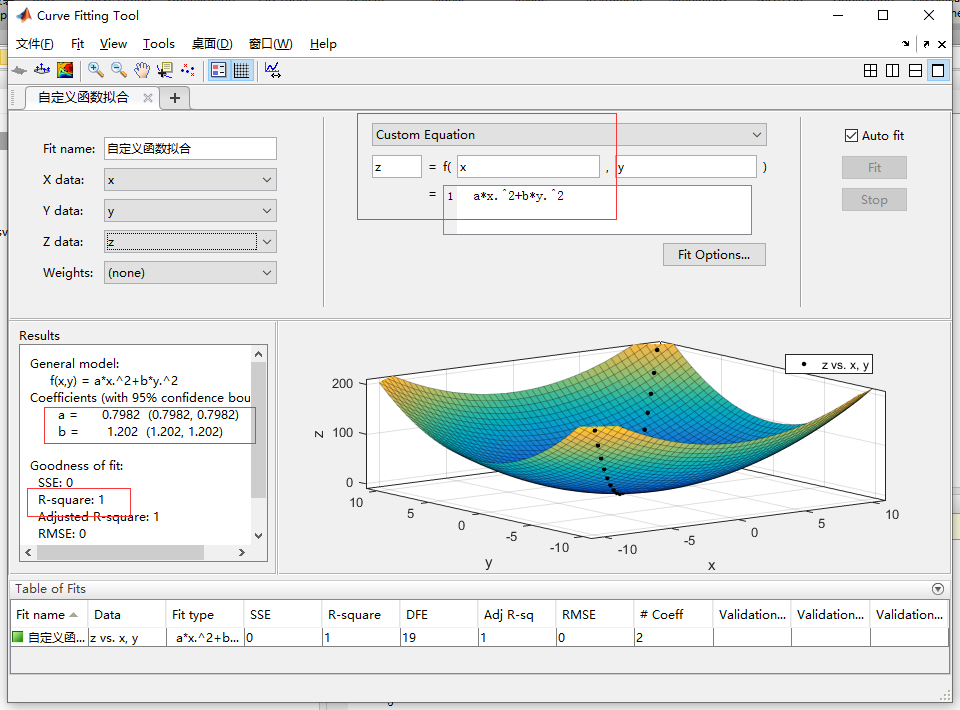x=-10:10;
y=-10:10;
z=x.^2+y.^2;
x_=-10:.1:10;
y_=-10:.1:10;



# 人口数据的不同拟合对比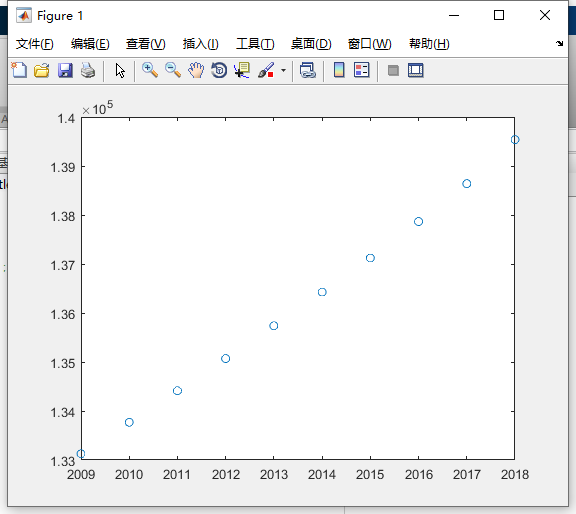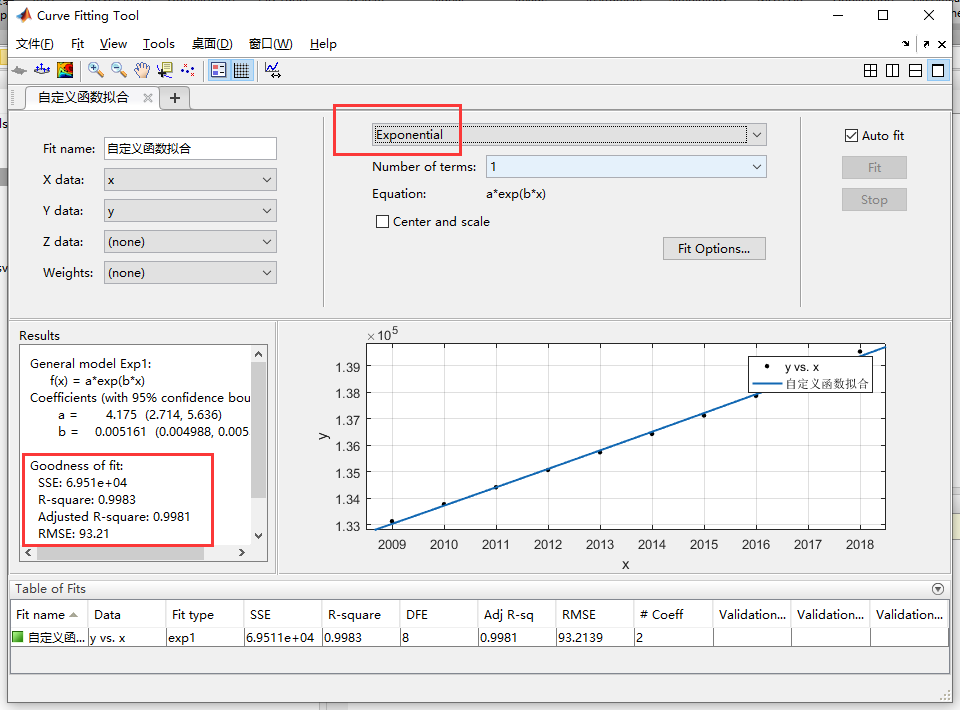傅里叶函数拟合（三角函数）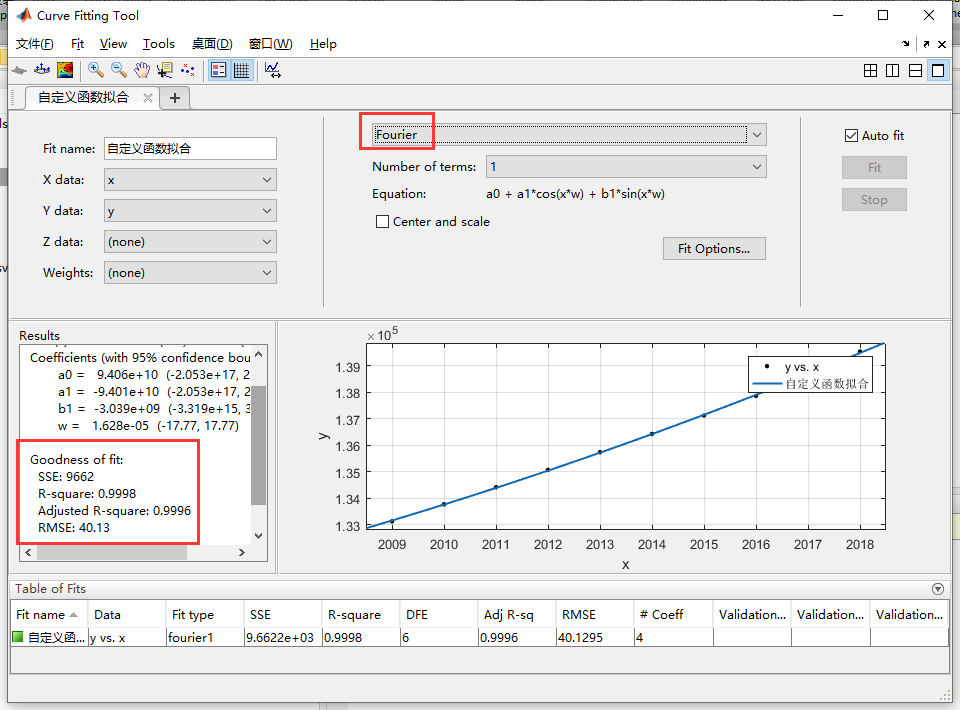高斯函数拟合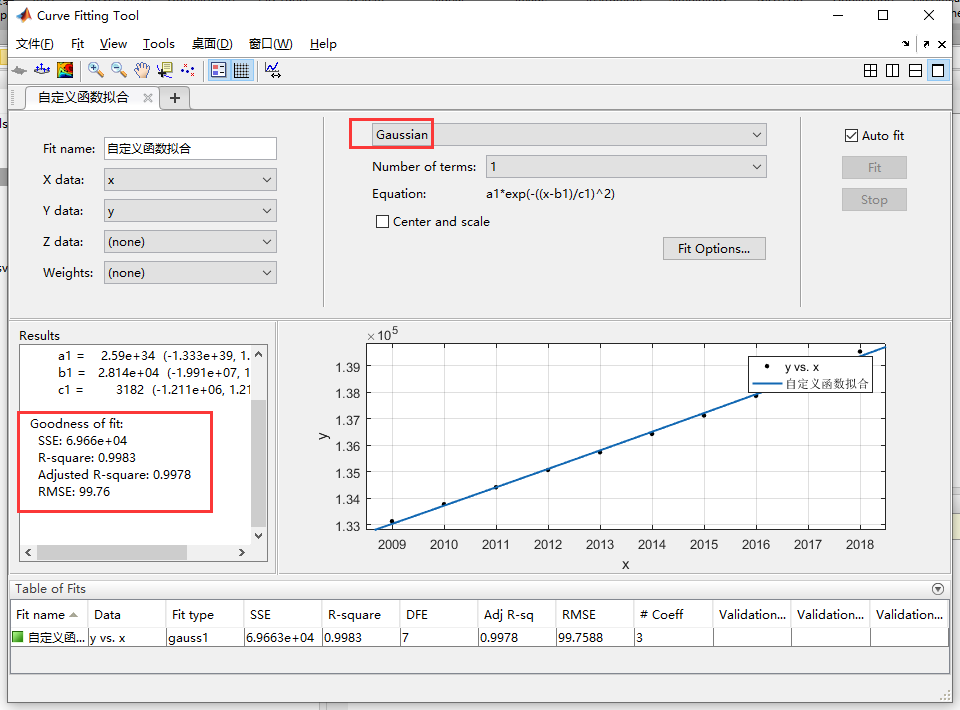线性插值拟合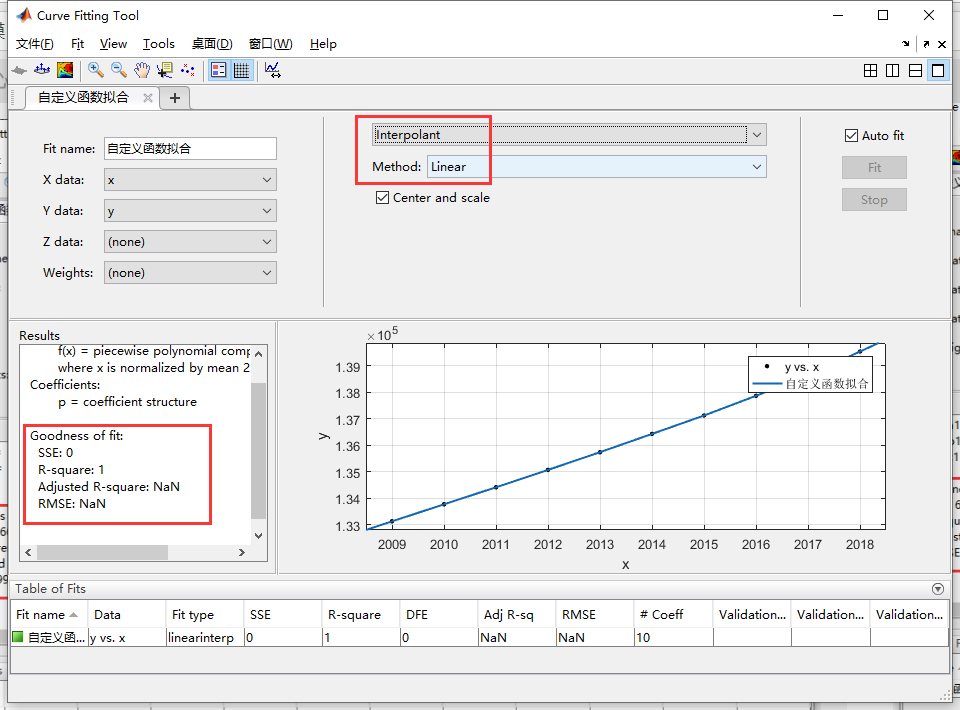最近邻插值拟合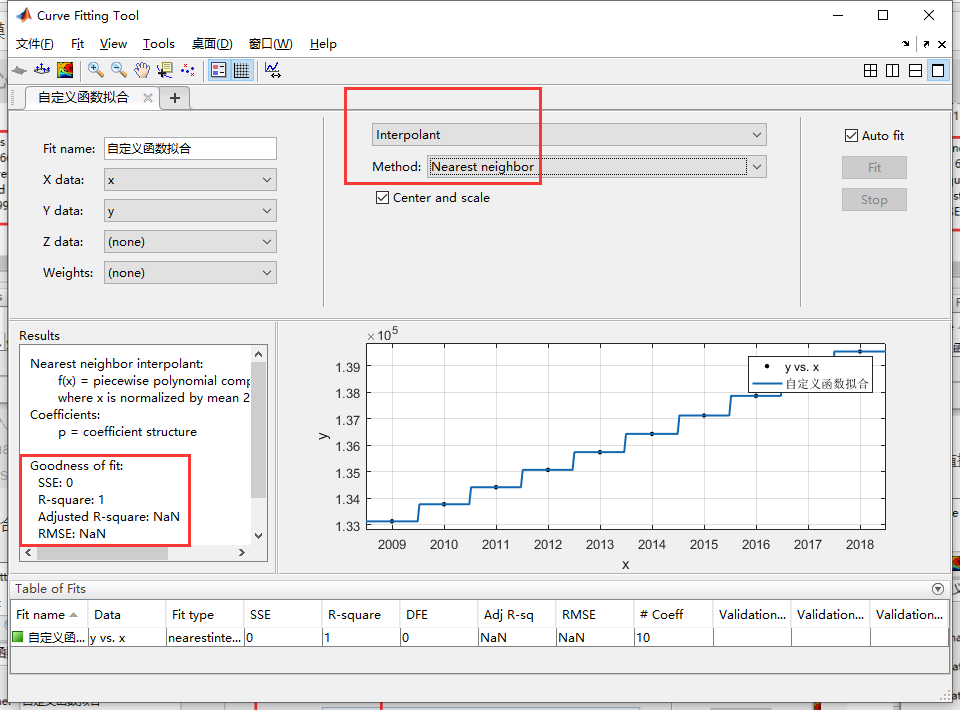保凹凸性（hermite ）拟合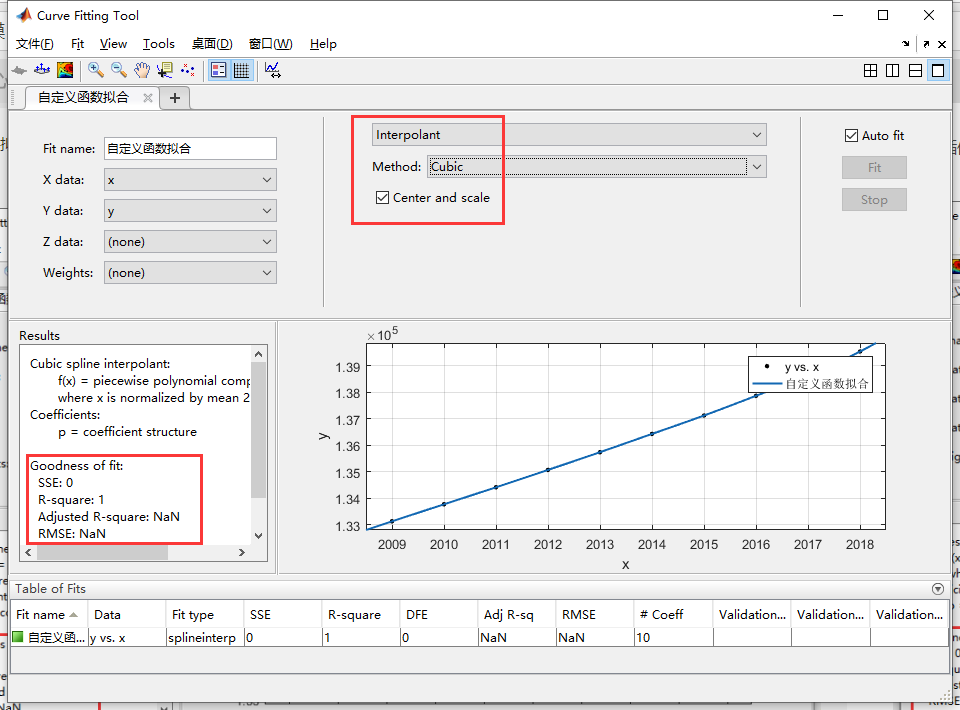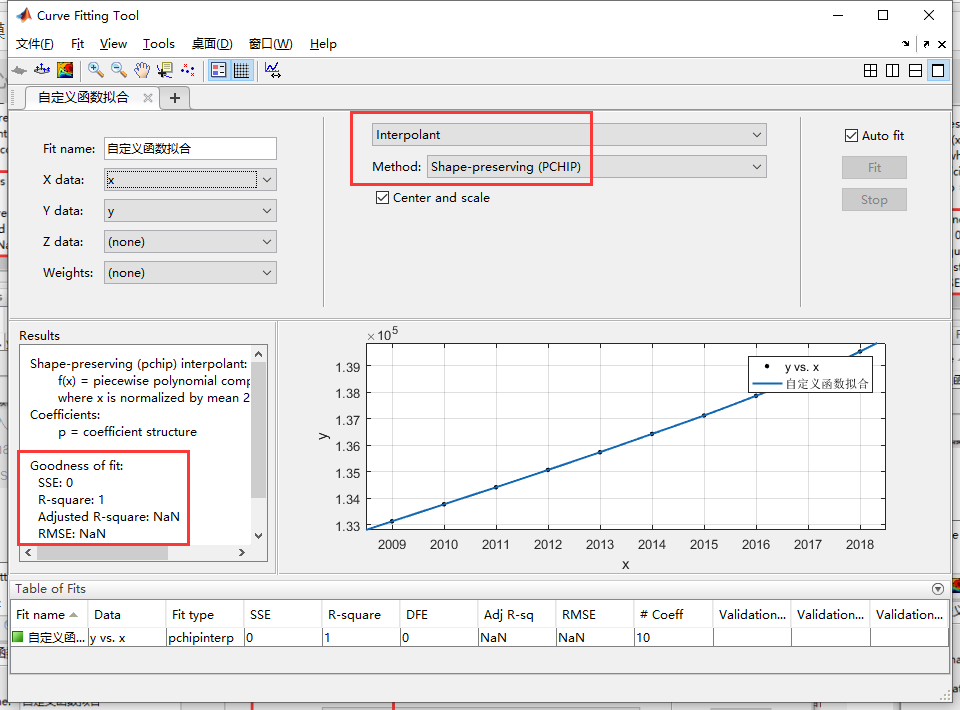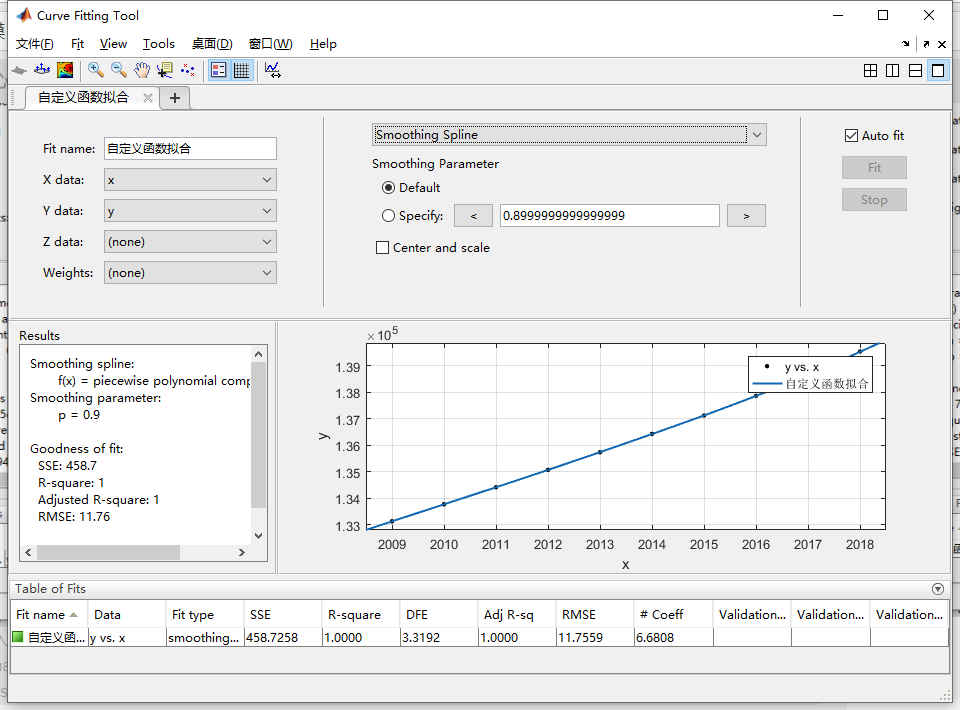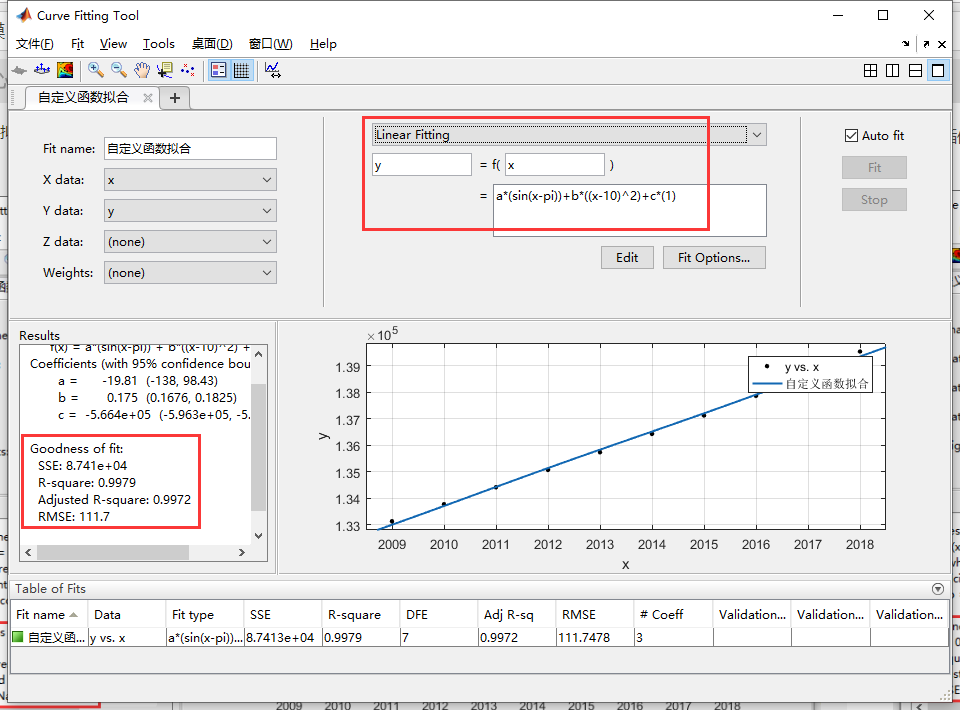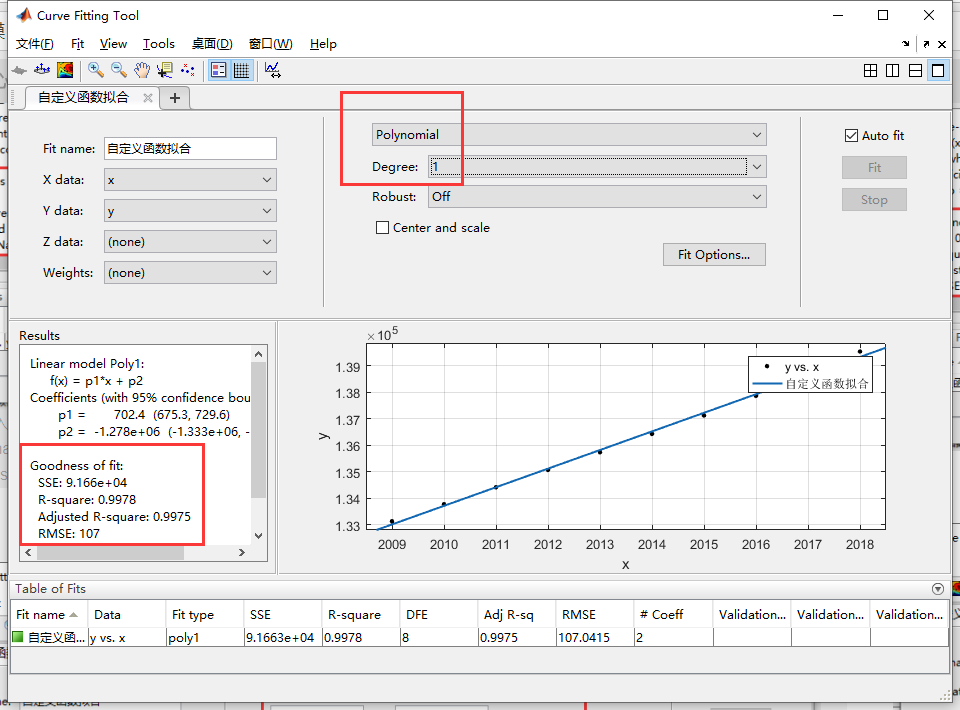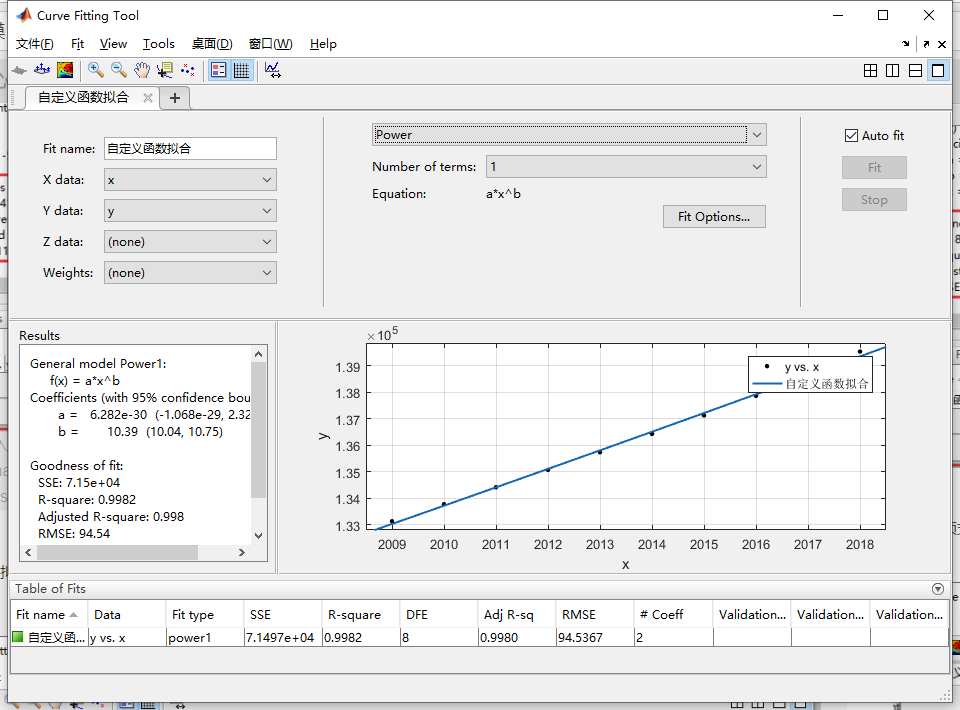01-28498
01-228214
10-096341
05-132万+
07-251万+
12-30
09-142501
05-141539
05-231万+
10-28
10-02
09-214432
02-253万+
06-162404
04-043355
03-162211
07-127229

### “相关推荐”对你有帮助么？

•非常没帮助
•没帮助
•一般
•有帮助
•非常有帮助被折叠的  条评论 为什么被折叠?到【灌水乐园】发言doubleslow;

¥2 ¥4 ¥6 ¥10 ¥20余额支付 (余额：-- )扫码支付获取中扫码支付点击重新获取扫码支付1.余额是钱包充值的虚拟货币，按照1:1的比例进行支付金额的抵扣。
2.余额无法直接购买下载，可以购买VIP、C币套餐、付费专栏及课程。余额充值